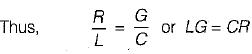Courses

# Test: Transmission Lines- 1

## 10 Questions MCQ Test Topicwise Question Bank for Electronics Engineering | Test: Transmission Lines- 1

Description
This mock test of Test: Transmission Lines- 1 for Electronics and Communication Engineering (ECE) helps you for every Electronics and Communication Engineering (ECE) entrance exam. This contains 10 Multiple Choice Questions for Electronics and Communication Engineering (ECE) Test: Transmission Lines- 1 (mcq) to study with solutions a complete question bank. The solved questions answers in this Test: Transmission Lines- 1 quiz give you a good mix of easy questions and tough questions. Electronics and Communication Engineering (ECE) students definitely take this Test: Transmission Lines- 1 exercise for a better result in the exam. You can find other Test: Transmission Lines- 1 extra questions, long questions & short questions for Electronics and Communication Engineering (ECE) on EduRev as well by searching above.
QUESTION: 1

### For a reciprocal transmission line, the three transmission parameters are given by A = 4, B = 7 and C = 5. The value of D is equal to

Solution:

Since the transmission line is reciprocal, therefore,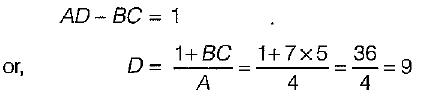QUESTION: 2

Solution:
QUESTION: 3

### In a transmission line terminated by characteristic impedance, Z0

Solution:

When a transmission line is terminated by an impedance Z, then reflection coefficient are: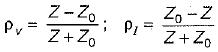Here, Z0 = Characteristic impedance of transmission line.
Since Z = Z0 therefore reflection co-efficient of voltage (pv) and current (pI) both will be zero, i.e there will be no reflection of incident wave.

QUESTION: 4

A symmetrical two-part network have

Solution:
QUESTION: 5

A pure resistance, RL when connected at the load end of a loss-less 100 Ω line produces a VSWR of 2. The RL would be

Solution: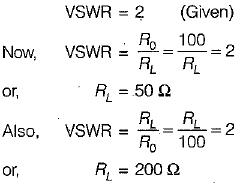QUESTION: 6

The reflection coefficient of a transmission line with a short-circuited load is

Solution:

reflection coefficient of voltage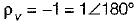and reflection coefficient of current,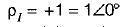Here, only option (b) matches the answer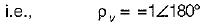QUESTION: 7

'The characteristic impedance Z0 of a transmission line is given by (where R, L, G, C are the unit length parameters)

Solution:

The characteristic impedance (Z0) of a transmission line is given by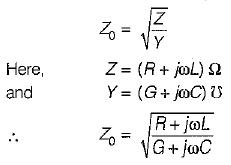QUESTION: 8

The standing wave ratio (VSWR) in terms of reflection coefficient ρ is given by

Solution:
QUESTION: 9

If a transmission line of length less than λ/4  is short circuited, it behaves as

Solution:
QUESTION: 10

A line becomes distortionless

Solution:

A distortionless transmission line is one in which the attenuation constant α is independent of frequency while the phase.constant β is linearly dependent on frequency.